# Test: Electrostatic Potential Energy

## 10 Questions MCQ Test Physics For JEE | Test: Electrostatic Potential Energy

Description
Attempt Test: Electrostatic Potential Energy | 10 questions in 10 minutes | Mock test for NEET preparation | Free important questions MCQ to study Physics For JEE for NEET Exam | Download free PDF with solutions
QUESTION: 1

### A particle of mass 1 kg and charge 1/3μC is projected towards a non conducting fixed spherical shell having the same charge uniformly distributed on its surface. The minimum initial velocity V0 of projection of particle required if the particle just grazes the shell is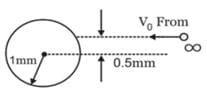Solution:

From conservation of angular momentum,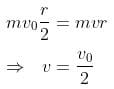from conservation of energy,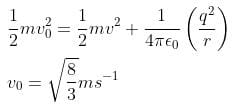QUESTION: 2

Solution:

Definition based

QUESTION: 3

### Negative mutual potential energy corresponds to attraction between two charges

Solution:

Definition based

QUESTION: 4

The amount of work done in moving a charge from one point to another along an equipotential line or surface charge is

Solution:

Since Potential difference between two points in equipotential surfaces is zero, the work done between two points in equipotential surface is also zero.

QUESTION: 5

In bringing an electron towards another electron, the electrostatic potential energy of the system

Solution:

Definition based

QUESTION: 6

The potential energy of a system containing only one point charge is

Solution:
QUESTION: 7

A proton is accelerated from rest through a potential of 500 volts. Its final kinetic energy is

Solution:

K = qV

QUESTION: 8

The electrostatic potential energy between two charges q1 and q2 separated by a distance by r is given by

Solution:

We know that energy required/gained = force x displacement
As F = kqQ / r2 and displacement is r
We get U = kQq /r
Where k = 1/4ϵ

QUESTION: 9

What is the electrostatic potential energy of a system containing two charges 40 nC and 60 nC at a distance of 36 cm?

Solution:

Definition based

*Answer can only contain numeric values
QUESTION: 10

Three point charges Q, +q and +q are placed at the vertices of a right-angled isosceles triangle as shown in the figure. If the net electrostaic energy of the configuration is zero, find the value Q\q is [Take √2 = 1.4]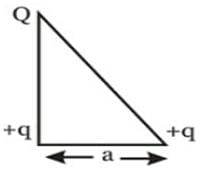Solution:

Net electrostatic energy of the configuration will be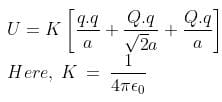Putting U = 0 we get,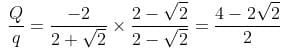-(2-√2) = -0.6Use Code STAYHOME200 and get INR 200 additional OFF Use Coupon Code